# NCERT Exemplar Class 11 Chemistry Solutions for Chapter 8 - Redox Reactions

## NCERT Exemplar Solutions Class 11 Chemistry Chapter 8 – Free PDF Download

NCERT Exemplar Chemistry Class 11 Chapter 8 Redox Reaction help students test their knowledge about Redox Reaction and learn about the different concepts related to it. The exemplar for Chapter 8 we are offering consists of the main questions covering all the topics in the NCERT Exemplar book. Along with textbook solutions, these solutions provide problems and worksheet that guides you in understanding the topic thoroughly. The set of solutions for NCERT Exemplar Cass 11 Chemistry Chapter Redox Reaction is available in the form of a PDF which is very beneficial as students can freely download it from our website and use it at any other time as resource material.

NCERT Exemplar Chemistry Class 11 Chapter 8 PDF has varieties of question including MCQ’S, fill in the blanks, match the following, short and long answer questions, worksheets, exercises, tips and tricks that are crucial for your Class 11 Chemistry examination and graduate entrance examinations. The questions and explanations have been prepared and solved by our top subject experts as per the latest CBSE Syllabus (2021-22). Before solving the questions let us understand what redox reaction is. An oxidation-reduction (redox) reaction is a type of chemical reaction that involves the transfer of electrons between two species.

### Download PDF of NCERT Exemplar for Class 11 Chemistry Chapter 8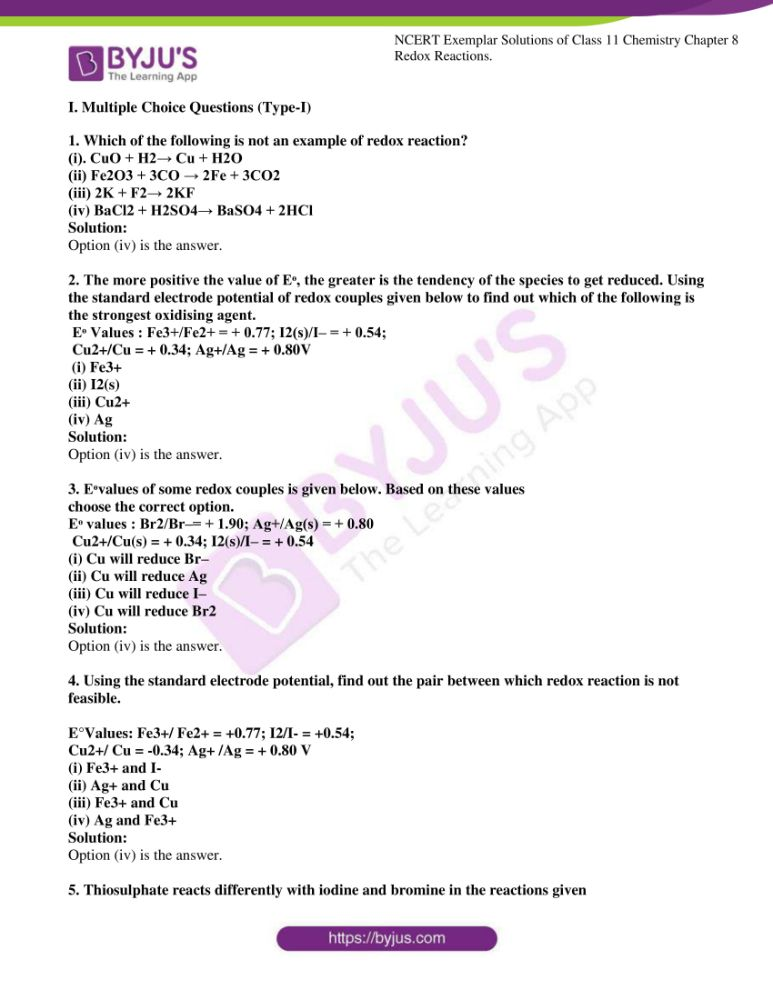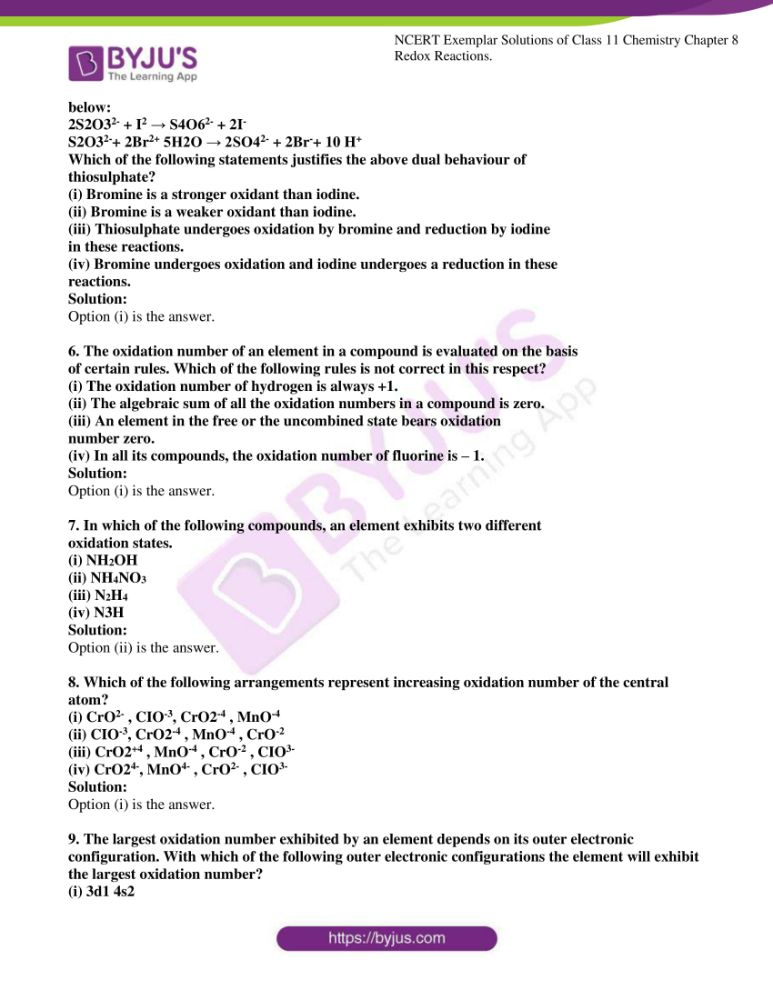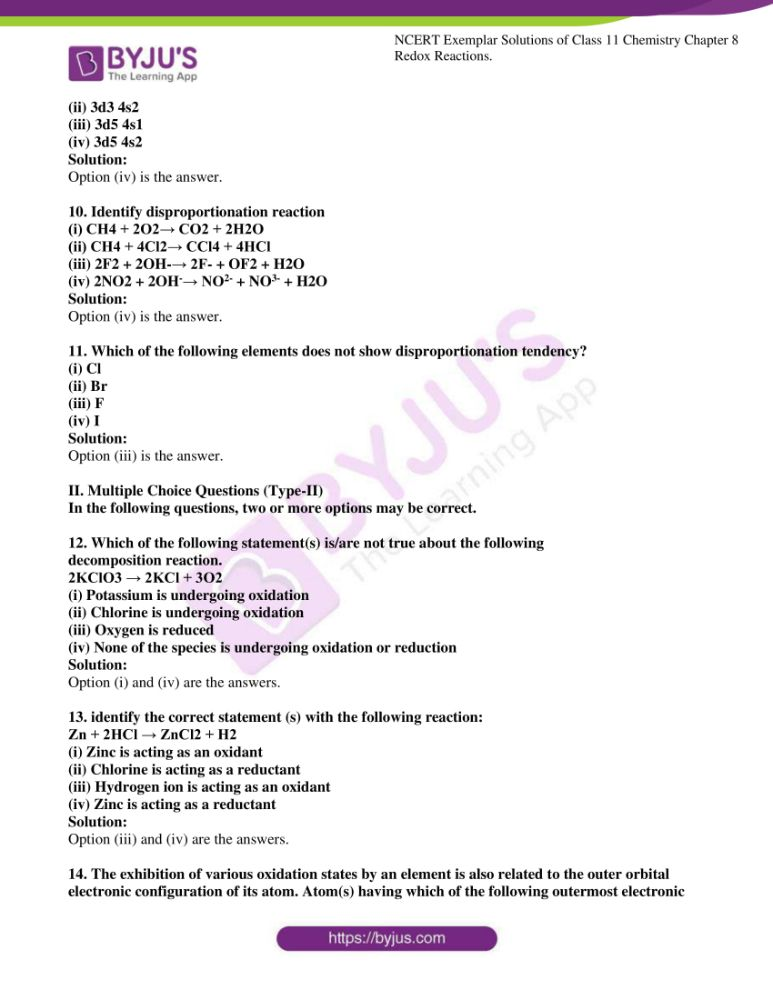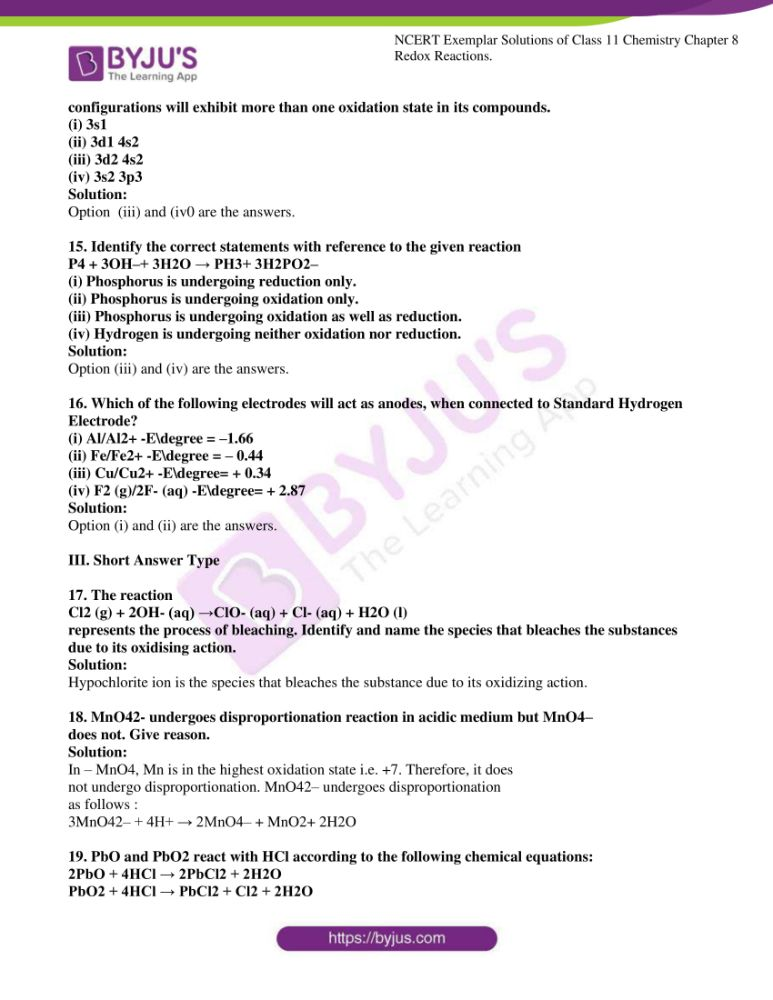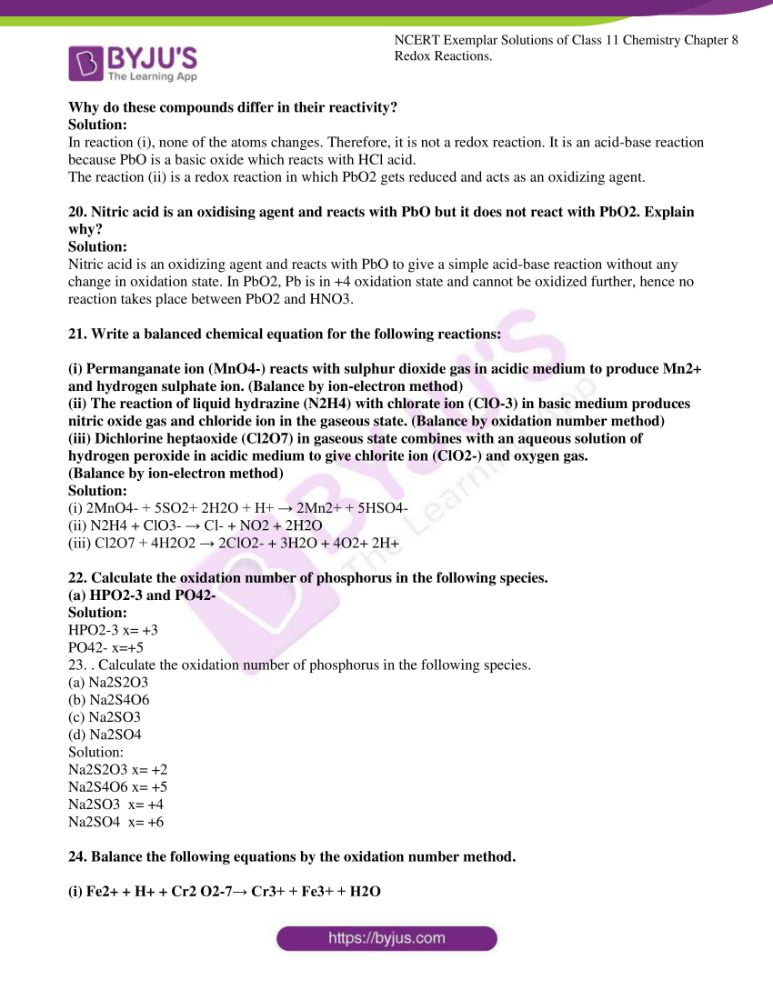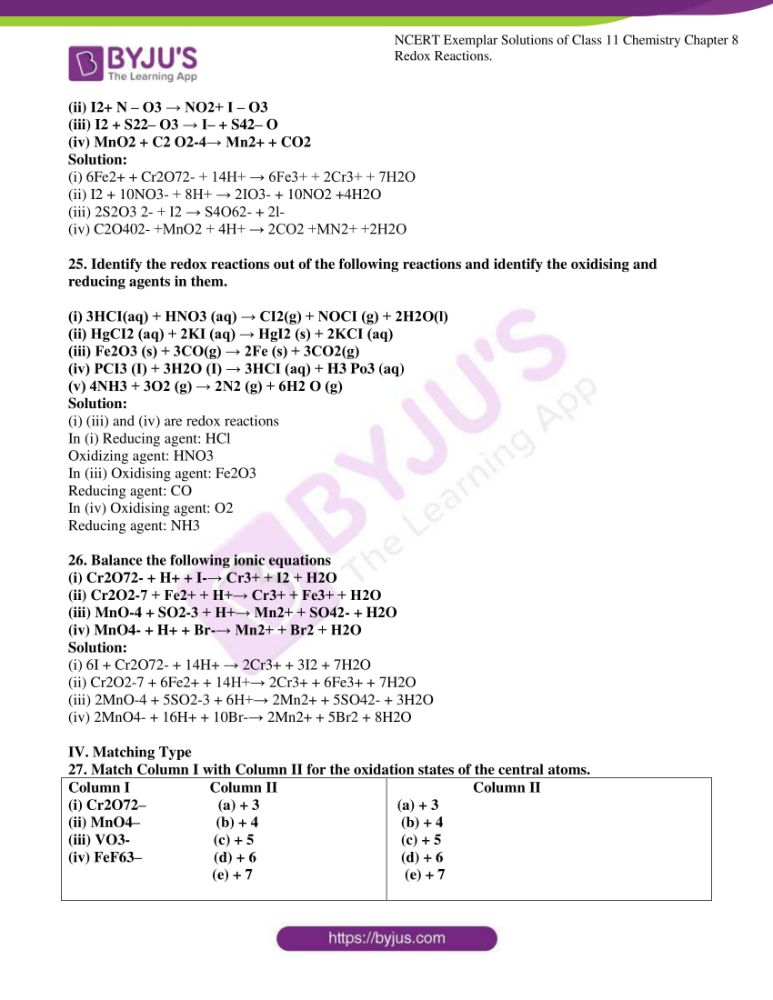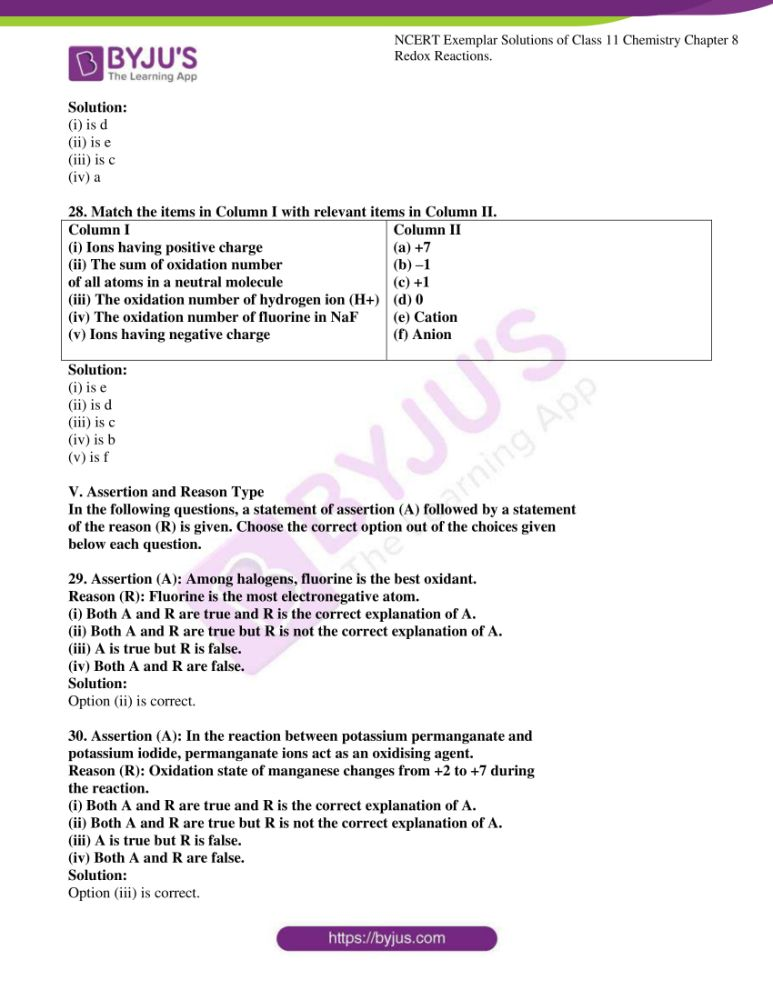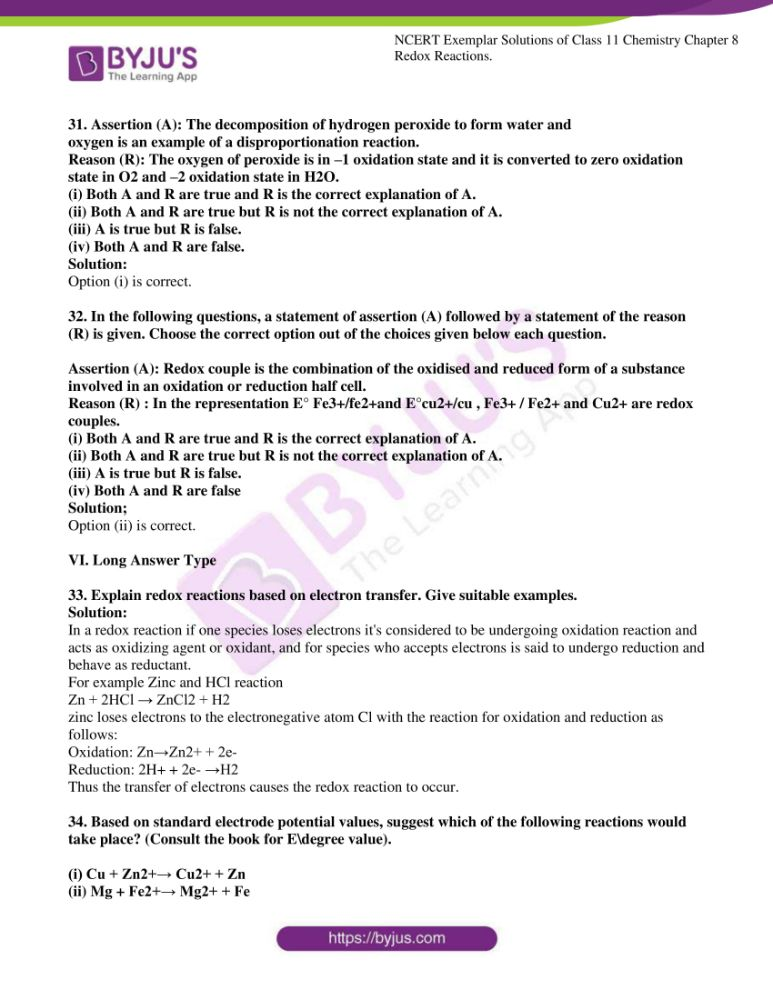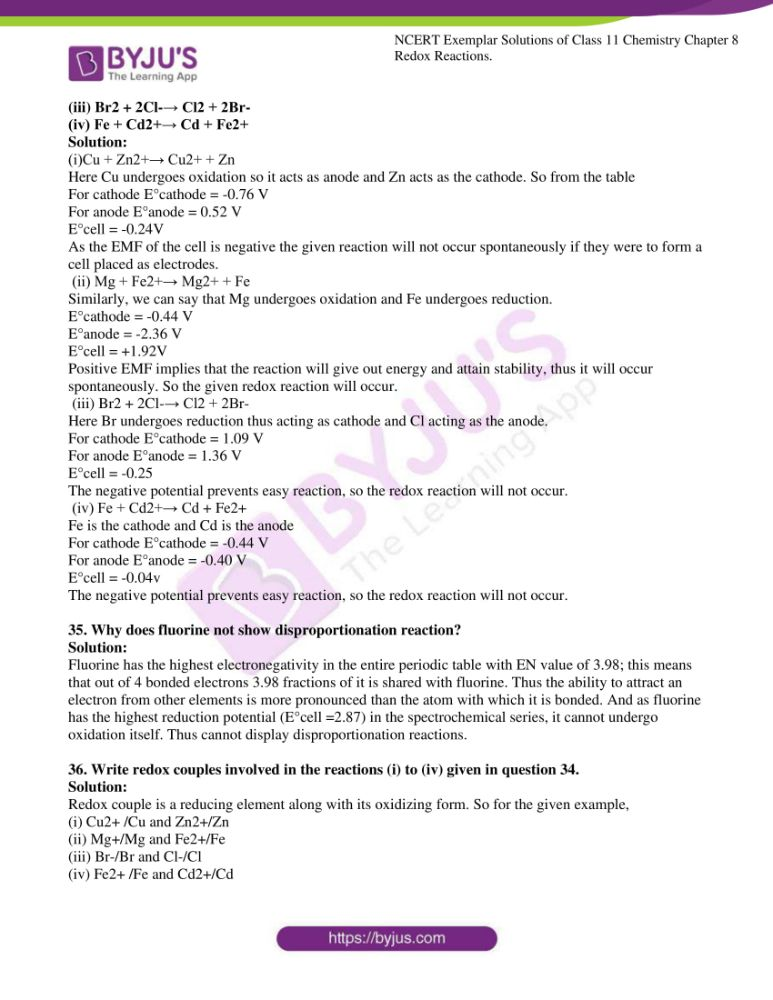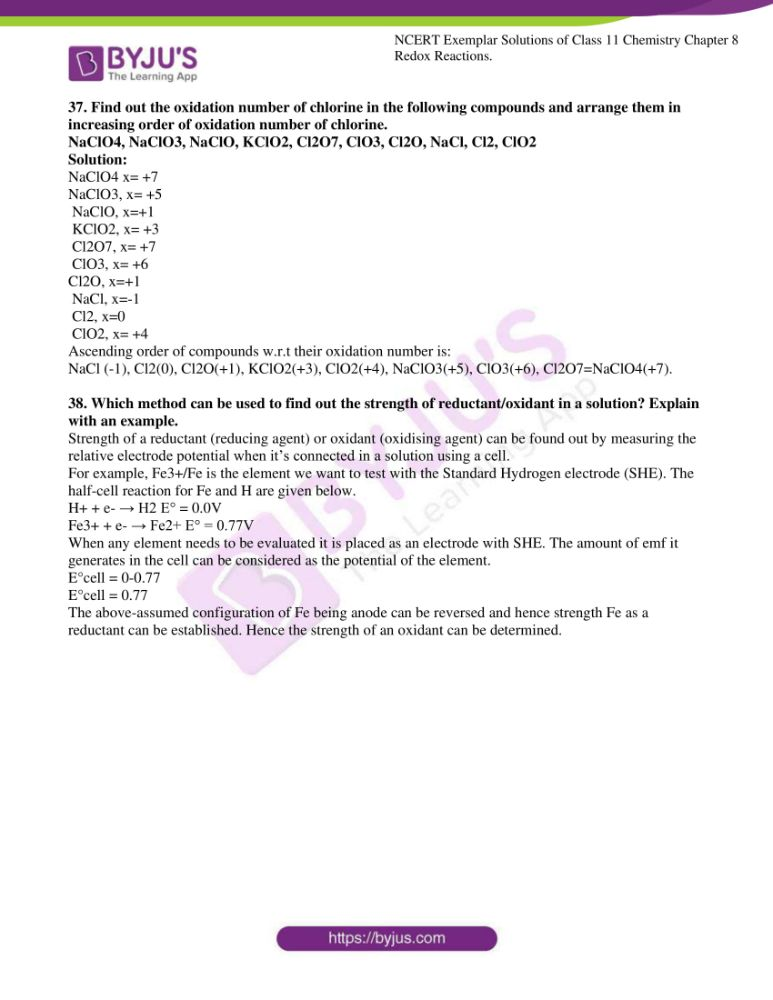### Access Ncert Exemplar For Class 11 Chemistry Chapter 8

I. Multiple Choice Questions (Type-I)

1. Which of the following is not an example of redox reaction?

(i). CuO + H2→ Cu + H2O

(ii) Fe2O3 + 3CO → 2Fe + 3CO2

(iii) 2K + F2→ 2KF

(iv) BaCl2 + H2SO4→ BaSO4 + 2HCl

Solution:

2. The more positive the value of Eᶱ, the greater is the tendency of the species to get reduced. Using the standard electrode potential of redox couples given below to find out which of the following is the strongest oxidising agent. Eᶱ Values : Fe3+/Fe2+ = + 0.77; I2(s)/I– = + 0.54;

Cu2+/Cu = + 0.34; Ag+/Ag = + 0.80V (i) Fe3+

(ii) I2(s)

(iii) Cu2+

(iv) Ag

Solution:

3. Eᶱvalues of some redox couples is given below. Based on these values

choose the correct option.

Eᶱ values : Br2/Br–= + 1.90; Ag+/Ag(s) = + 0.80

Cu2+/Cu(s) = + 0.34; I2(s)/I– = + 0.54

(i) Cu will reduce Br–

(ii) Cu will reduce Ag

(iii) Cu will reduce I–

(iv) Cu will reduce Br2

Solution:

4. Using the standard electrode potential, find out the pair between which redox reaction is not feasible.

E°Values: Fe3+/ Fe2+ = +0.77; I2/I- = +0.54;

Cu2+/ Cu = -0.34; Ag+ /Ag = + 0.80 V

(i) Fe3+ and I-

(ii) Ag+ and Cu

(iii) Fe3+ and Cu

(iv) Ag and Fe3+

Solution:

5. Thiosulphate reacts differently with iodine and bromine in the reactions given

below:

2S2O32- + I2 → S4O62- + 2I

S2O32-+ 2Br2+ 5H2O → 2SO42- + 2Br+ 10 H+

Which of the following statements justifies the above dual behaviour of

thiosulphate?

(i) Bromine is a stronger oxidant than iodine.

(ii) Bromine is a weaker oxidant than iodine.

(iii) Thiosulphate undergoes oxidation by bromine and reduction by iodine

in these reactions.

(iv) Bromine undergoes oxidation and iodine undergoes a reduction in these

reactions.

Solution:

6. The oxidation number of an element in a compound is evaluated on the basis

of certain rules. Which of the following rules is not correct in this respect?

(i) The oxidation number of hydrogen is always +1.

(ii) The algebraic sum of all the oxidation numbers in a compound is zero.

(iii) An element in the free or the uncombined state bears oxidation

number zero.

(iv) In all its compounds, the oxidation number of fluorine is – 1.Solution:

7. In which of the following compounds, an element exhibits two different

oxidation states.

(i) NH2OH

(ii) NH4NO3

(iii) N2H4

(iv) N3H

Solution:

8. Which of the following arrangements represent increasing oxidation number of the central atom?

(i) CrO2- , CIO-3, CrO2-4 , MnO-4

(ii) CIO-3, CrO2-4 , MnO-4 , CrO-2

(iii) CrO2+4 , MnO-4 , CrO-2 , CIO3-

(iv) CrO24-, MnO4- , CrO2- , CIO3-

Solution:

9. The largest oxidation number exhibited by an element depends on its outer electronic configuration. With which of the following outer electronic configurations the element will exhibit the largest oxidation number?

(i) 3d1 4s2

(ii) 3d3 4s2

(iii) 3d5 4s1

(iv) 3d5 4s2

Solution:

10. Identify disproportionation reaction

(i) CH4 + 2O2→ CO2 + 2H2O

(ii) CH4 + 4Cl2→ CCl4 + 4HCl

(iii) 2F2 + 2OH-→ 2F- + OF2 + H2O

(iv) 2NO2 + 2OH→ NO2- + NO3- + H2O

Solution:

11. Which of the following elements does not show disproportionation tendency?

(i) Cl

(ii) Br

(iii) F

(iv) ISolution:

II. Multiple Choice Questions (Type-II)

In the following questions, two or more options may be correct.

12. Which of the following statement(s) is/are not true about the following

decomposition reaction.

2KClO3 → 2KCl + 3O2

(i) Potassium is undergoing oxidation

(ii) Chlorine is undergoing oxidation

(iii) Oxygen is reduced

(iv) None of the species is undergoing oxidation or reduction

Solution:

Option (i) and (iv) are the answers.

13. identify the correct statement (s) with the following reaction:

Zn + 2HCl → ZnCl2 + H2

(i) Zinc is acting as an oxidant

(ii) Chlorine is acting as a reductant

(iii) Hydrogen ion is acting as an oxidant

(iv) Zinc is acting as a reductant

Solution:

Option (iii) and (iv) are the answers.

14. The exhibition of various oxidation states by an element is also related to the outer orbital electronic configuration of its atom. Atom(s) having which of the following outermost electronic configurations will exhibit more than one oxidation state in its compounds.

(i) 3s1

(ii) 3d1 4s2

(iii) 3d2 4s2

(iv) 3s2 3p3

Solution:

Option (iii) and (iv0 are the answers.

15. Identify the correct statements with reference to the given reaction

P4 + 3OH–+ 3H2O → PH3+ 3H2PO2–

(i) Phosphorus is undergoing reduction only.

(ii) Phosphorus is undergoing oxidation only.

(iii) Phosphorus is undergoing oxidation as well as reduction.

(iv) Hydrogen is undergoing neither oxidation nor reduction.

Solution:

Option (iii) and (iv) are the answers.

16. Which of the following electrodes will act as anodes, when connected to Standard Hydrogen Electrode?

(i) Al/Al2+ -E\degree = –1.66

(ii) Fe/Fe2+ -E\degree = – 0.44

(iii) Cu/Cu2+ -E\degree= + 0.34

(iv) F2 (g)/2F- (aq) -E\degree= + 2.87

Solution:

Option (i) and (ii) are the answers.

17. The reaction

Cl2 (g) + 2OH- (aq) →ClO- (aq) + Cl- (aq) + H2O (l)

represents the process of bleaching. Identify and name the species that bleaches the substances due to its oxidising action.

Solution:

Hypochlorite ion is the species that bleaches the substance due to its oxidizing action.

18. MnO42- undergoes disproportionation reaction in acidic medium but MnO4–

does not. Give reason.Solution:

In – MnO4, Mn is in the highest oxidation state i.e. +7. Therefore, it does

not undergo disproportionation. MnO42– undergoes disproportionation

as follows :

3MnO42– + 4H+ → 2MnO4– + MnO2+ 2H2O

19. PbO and PbO2 react with HCl according to the following chemical equations:

2PbO + 4HCl → 2PbCl2 + 2H2O

PbO2 + 4HCl → PbCl2 + Cl2 + 2H2O

Why do these compounds differ in their reactivity?

Solution:

In reaction (i), none of the atoms changes. Therefore, it is not a redox reaction. It is an acid-base reaction because PbO is a basic oxide which reacts with HCl acid.

The reaction (ii) is a redox reaction in which PbO2 gets reduced and acts as an oxidizing agent.

20. Nitric acid is an oxidising agent and reacts with PbO but it does not react with PbO2. Explain why?

Solution:

Nitric acid is an oxidizing agent and reacts with PbO to give a simple acid-base reaction without any change in oxidation state. In PbO2, Pb is in +4 oxidation state and cannot be oxidized further, hence no reaction takes place between PbO2 and HNO3.

21. Write a balanced chemical equation for the following reactions:

(i) Permanganate ion (MnO4-) reacts with sulphur dioxide gas in acidic medium to produce Mn2+ and hydrogen sulphate ion. (Balance by ion-electron method)

(ii) The reaction of liquid hydrazine (N2H4) with chlorate ion (ClO-3) in basic medium produces nitric oxide gas and chloride ion in the gaseous state. (Balance by oxidation number method)

(iii) Dichlorine heptaoxide (Cl2O7) in gaseous state combines with an aqueous solution of hydrogen peroxide in acidic medium to give chlorite ion (ClO2-) and oxygen gas.

(Balance by ion-electron method)

Solution:

(i) 2MnO4- + 5SO2+ 2H2O + H+ → 2Mn2+ + 5HSO4-

(ii) N2H4 + ClO3- → Cl- + NO2 + 2H2O

(iii) Cl2O7 + 4H2O2 → 2ClO2- + 3H2O + 4O2+ 2H+

22. Calculate the oxidation number of phosphorus in the following species.

(a) HPO2-3 and PO42-

Solution:

HPO2-3 x= +3

PO42- x=+5

23. . Calculate the oxidation number of phosphorus in the following species.

(a) Na2S2O3

(b) Na2S4O6

(c) Na2SO3

(d) Na2SO4

Solution:

Na2S2O3 x= +2

Na2S4O6 x= +5

Na2SO3 x= +4

Na2SO4 x= +6

24. Balance the following equations by the oxidation number method.

(i) Fe2+ + H+ + Cr2 O2-7→ Cr3+ + Fe3+ + H2O

(ii) I2+ N – O3 → NO2+ I – O3

(iii) I2 + S22– O3 → I– + S42– O

(iv) MnO2 + C2 O2-4→ Mn2+ + CO2

Solution:

(i) 6Fe2+ + Cr2O72- + 14H+ → 6Fe3+ + 2Cr3+ + 7H2O

(ii) I2 + 10NO3- + 8H+ → 2IO3- + 10NO2 +4H2O

(iii) 2S2O3 2- + I2 → S4O62- + 2l-

(iv) C2O402- +MnO2 + 4H+ → 2CO2 +MN2+ +2H2O

25. Identify the redox reactions out of the following reactions and identify the oxidising and reducing agents in them.

(i) 3HCI(aq) + HNO3 (aq) → CI2(g) + NOCI (g) + 2H2O(l)

(ii) HgCI2 (aq) + 2KI (aq) → HgI2 (s) + 2KCI (aq)

(iii) Fe2O3 (s) + 3CO(g) → 2Fe (s) + 3CO2(g)

(iv) PCI3 (I) + 3H2O (I) → 3HCI (aq) + H3 Po3 (aq)

(v) 4NH3 + 3O2 (g) → 2N2 (g) + 6H2 O (g)

Solution:

(i) (iii) and (iv) are redox reactions

In (i) Reducing agent: HCl

Oxidizing agent: HNO3

In (iii) Oxidising agent: Fe2O3

Reducing agent: CO

In (iv) Oxidising agent: O2

Reducing agent: NH3

26. Balance the following ionic equations

(i) Cr2O72- + H+ + I-→ Cr3+ + I2 + H2O

(ii) Cr2O2-7 + Fe2+ + H+→ Cr3+ + Fe3+ + H2O

(iii) MnO-4 + SO2-3 + H+→ Mn2+ + SO42- + H2O

(iv) MnO4- + H+ + Br-→ Mn2+ + Br2 + H2O

Solution:

(i) 6I + Cr2O72- + 14H+ → 2Cr3+ + 3I2 + 7H2O

(ii) Cr2O2-7 + 6Fe2+ + 14H+→ 2Cr3+ + 6Fe3+ + 7H2O

(iii) 2MnO-4 + 5SO2-3 + 6H+→ 2Mn2+ + 5SO42- + 3H2O

(iv) 2MnO4- + 16H+ + 10Br-→ 2Mn2+ + 5Br2 + 8H2O

IV. Matching Type

27. Match Column I with Column II for the oxidation states of the central atoms.

 Column I Column II (i) Cr2O72– (a) + 3 (ii) MnO4– (b) + 4 (iii) VO3- (c) + 5 (iv) FeF63– (d) + 6 (e) + 7 Column II (a) + 3 (b) + 4 (c) + 5 (d) + 6 (e) + 7

Solution:

(i) is d

(ii) is e

(iii) is c

(iv) a

28. Match the items in Column I with relevant items in Column II.

 Column I (i) Ions having positive charge (ii) The sum of oxidation number of all atoms in a neutral molecule (iii) The oxidation number of hydrogen ion (H+) (iv) The oxidation number of fluorine in NaF (v) Ions having negative charge Column II (a) +7 (b) –1 (c) +1 (d) 0 (e) Cation (f) Anion

Solution:

(i) is e

(ii) is d

(iii) is c

(iv) is b

(v) is f

V. Assertion and Reason Type

In the following questions, a statement of assertion (A) followed by a statement

of the reason (R) is given. Choose the correct option out of the choices given

below each question.

29. Assertion (A): Among halogens, fluorine is the best oxidant.

Reason (R): Fluorine is the most electronegative atom.

(i) Both A and R are true and R is the correct explanation of A.

(ii) Both A and R are true but R is not the correct explanation of A.

(iii) A is true but R is false.

(iv) Both A and R are false.

Solution:

Option (ii) is correct.

30. Assertion (A): In the reaction between potassium permanganate and

potassium iodide, permanganate ions act as an oxidising agent.

Reason (R): Oxidation state of manganese changes from +2 to +7 during

the reaction.

(i) Both A and R are true and R is the correct explanation of A.

(ii) Both A and R are true but R is not the correct explanation of A.

(iii) A is true but R is false.

(iv) Both A and R are false.

Solution:

Option (iii) is correct.

31. Assertion (A): The decomposition of hydrogen peroxide to form water and

oxygen is an example of a disproportionation reaction.

Reason (R): The oxygen of peroxide is in –1 oxidation state and it is converted to zero oxidation state in O2 and –2 oxidation state in H2O.

(i) Both A and R are true and R is the correct explanation of A.

(ii) Both A and R are true but R is not the correct explanation of A.

(iii) A is true but R is false.

(iv) Both A and R are false.

Solution:

Option (i) is correct.

32. In the following questions, a statement of assertion (A) followed by a statement of the reason (R) is given. Choose the correct option out of the choices given below each question.

Assertion (A): Redox couple is the combination of the oxidised and reduced form of a substance involved in an oxidation or reduction half cell.

Reason (R) : In the representation E° Fe3+/fe2+and E°cu2+/cu , Fe3+ / Fe2+ and Cu2+ are redox couples.

(i) Both A and R are true and R is the correct explanation of A.

(ii) Both A and R are true but R is not the correct explanation of A.

(iii) A is true but R is false.

(iv) Both A and R are false

Solution;

Option (ii) is correct.

33. Explain redox reactions based on electron transfer. Give suitable examples.

Solution:

In a redox reaction if one species loses electrons it’s considered to be undergoing oxidation reaction and acts as oxidizing agent or oxidant, and for species who accepts electrons is said to undergo reduction and behave as reductant.

For example Zinc and HCl reaction

Zn + 2HCl → ZnCl2 + H2

zinc loses electrons to the electronegative atom Cl with the reaction for oxidation and reduction as follows:

Oxidation: Zn→Zn2+ + 2e-

Reduction: 2H+ + 2e- →H2

Thus the transfer of electrons causes the redox reaction to occur.

34. Based on standard electrode potential values, suggest which of the following reactions would take place? (Consult the book for E\degree value).

(i) Cu + Zn2+→ Cu2+ + Zn

(ii) Mg + Fe2+→ Mg2+ + Fe

(iii) Br2 + 2Cl-→ Cl2 + 2Br-

(iv) Fe + Cd2+→ Cd + Fe2+

Solution:

(i)Cu + Zn2+→ Cu2+ + Zn

Here Cu undergoes oxidation so it acts as anode and Zn acts as the cathode. So from the table

For cathode E°cathode = -0.76 V

For anode E°anode = 0.52 V

E°cell = -0.24V

As the EMF of the cell is negative the given reaction will not occur spontaneously if they were to form a cell placed as electrodes.

(ii) Mg + Fe2+→ Mg2+ + Fe

Similarly, we can say that Mg undergoes oxidation and Fe undergoes reduction.

E°cathode = -0.44 V

E°anode = -2.36 V

E°cell = +1.92V

Positive EMF implies that the reaction will give out energy and attain stability, thus it will occur spontaneously. So the given redox reaction will occur.

(iii) Br2 + 2Cl-→ Cl2 + 2Br-

Here Br undergoes reduction thus acting as cathode and Cl acting as the anode.

For cathode E°cathode = 1.09 V

For anode E°anode = 1.36 V

E°cell = -0.25

The negative potential prevents easy reaction, so the redox reaction will not occur.

(iv) Fe + Cd2+→ Cd + Fe2+

Fe is the cathode and Cd is the anode

For cathode E°cathode = -0.44 V

For anode E°anode = -0.40 V

E°cell = -0.04v

The negative potential prevents easy reaction, so the redox reaction will not occur.

35. Why does fluorine not show disproportionation reaction?

Solution:

Fluorine has the highest electronegativity in the entire periodic table with EN value of 3.98; this means that out of 4 bonded electrons 3.98 fractions of it is shared with fluorine. Thus the ability to attract an electron from other elements is more pronounced than the atom with which it is bonded. And as fluorine has the highest reduction potential (E°cell =2.87) in the spectrochemical series, it cannot undergo oxidation itself. Thus cannot display disproportionation reactions.

36. Write redox couples involved in the reactions (i) to (iv) given in question 34.

Solution:

Redox couple is a reducing element along with its oxidizing form. So for the given example,

(i) Cu2+ /Cu and Zn2+/Zn

(ii) Mg+/Mg and Fe2+/Fe

(iii) Br-/Br and Cl-/Cl

(iv) Fe2+ /Fe and Cd2+/Cd

37. Find out the oxidation number of chlorine in the following compounds and arrange them in increasing order of oxidation number of chlorine.

NaClO4, NaClO3, NaClO, KClO2, Cl2O7, ClO3, Cl2O, NaCl, Cl2, ClO2

Solution:

NaClO4 x= +7

NaClO3, x= +5

NaClO, x=+1

KClO2, x= +3

Cl2O7, x= +7

ClO3, x= +6

Cl2O, x=+1

NaCl, x=-1

Cl2, x=0

ClO2, x= +4

Ascending order of compounds w.r.t their oxidation number is:

NaCl (-1), Cl2(0), Cl2O(+1), KClO2(+3), ClO2(+4), NaClO3(+5), ClO3(+6), Cl2O7=NaClO4(+7).

38. Which method can be used to find out the strength of reductant/oxidant in a solution? Explain with an example.

Strength of a reductant (reducing agent) or oxidant (oxidising agent) can be found out by measuring the relative electrode potential when it’s connected in a solution using a cell.

For example, Fe3+/Fe is the element we want to test with the Standard Hydrogen electrode (SHE). The half-cell reaction for Fe and H are given below.

H+ + e- → H2 E° = 0.0V

Fe3+ + e- → Fe2+ E° = 0.77V

When any element needs to be evaluated it is placed as an electrode with SHE. The amount of emf it generates in the cell can be considered as the potential of the element.

E°cell = 0-0.77

E°cell = 0.77

The above-assumed configuration of Fe being anode can be reversed and hence strength Fe as a reductant can be established. Hence the strength of an oxidant can be determined.

#### Topics of Class 11 Chemistry Chapter 8 Redox Reactions

1. Classical Idea Of Redox Reactions – Oxidation And Reduction Reactions
2. Redox Reactions In Terms Of Electron Transfer Reactions
1. Competitive Electron Transfer Reactions
3. Oxidation Number
1. Types Of Redox Reactions
2. Balancing Of Redox Reactions
3. Redox Reactions As The Basis For Titrations
4. Limitations Of Concept Of Oxidation Number
4. Redox Reactions And Electrode Processes.

The topics given in Class 11 syllabus are the basics to the topics to be taught in Class 12th. Students are advised to go through this NCERT Exemplars to determine their strengths and weaknesses and plan their study accordingly. Along with NCERT questions, students should try to solve the previous year questions and sample papers to get acquainted with the latest exam pattern and marking scheme.

## Frequently Asked Questions on NCERT Exemplar Solutions for Class 11 Chemistry Chapter 8

### Will I be able to get my doubts cleared using the NCERT Exemplar Solutions for Class 11 Chemistry Chapter 8?

Students who are not able to get their doubts cleared during class hours can make use of the NCERT Exemplar Solutions at BYJU’S. The experts frame the solutions with utmost care with the main objective of helping students with their board exam preparation. To score good marks in Chemistry, learning the concepts on a daily basis is very much important. The concepts are explained in an interactive manner to help students grasp them and perform well in the board exams.

### What are the topics covered under Chapter 8 of NCERT Exemplar Solutions for Class 11 Chemistry in the board exams?

Topics of Class 11 Chemistry Chapter 8 Redox Reactions are
1. Classical Idea Of Redox Reactions – Oxidation And Reduction Reactions
2. Redox Reactions In Terms Of Electron Transfer Reactions
3. Competitive Electron Transfer Reactions
4. Oxidation Number
5. Types Of Redox Reactions
6. Balancing Of Redox Reactions
7. Redox Reactions As The Basis For Titrations
8. Limitations Of Concept Of Oxidation Number
9. Redox Reactions And Electrode Processes.
To score full marks in this chapter, students are advised to solve the textbook questions using the solutions available at BYJU’S. The solutions of this chapter are framed by expert faculty having vast experience in the relevant subject. They create answers which are concept focused rather than question focused to build a strong foundation of basic concepts which are important from the exam perspective.

### Why should I refer to the NCERT Exemplar Solutions for Class 11 Chemistry Chapter 8 from BYJU’S?

You should refer to the NCERT Exemplar Solutions for Class 11 Chemistry Chapter 8 from BYJU’S to understand the concepts easily. The solutions are framed by the teachers based on the understanding abilities of students. Pictorial representation of certain topics improves visual learning skills among students which helps them to grasp the concepts. NCERT Exemplar Solutions are created in a stepwise manner in order to meet the CBSE standards. Every year the solutions are updated based on the changes occurring in the CBSE syllabus.